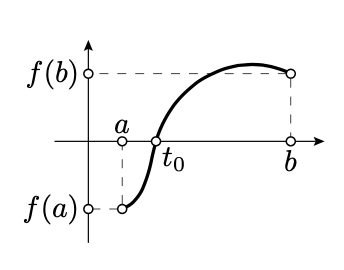$$\newcommand{\id}{\mathrm{id}}$$ $$\newcommand{\Span}{\mathrm{span}}$$ $$\newcommand{\kernel}{\mathrm{null}\,}$$ $$\newcommand{\range}{\mathrm{range}\,}$$ $$\newcommand{\RealPart}{\mathrm{Re}}$$ $$\newcommand{\ImaginaryPart}{\mathrm{Im}}$$ $$\newcommand{\Argument}{\mathrm{Arg}}$$ $$\newcommand{\norm}{\| #1 \|}$$ $$\newcommand{\inner}{\langle #1, #2 \rangle}$$ $$\newcommand{\Span}{\mathrm{span}}$$

# 3.2: Intermediate Value Theorem

$$\newcommand{\vecs}{\overset { \rightharpoonup} {\mathbf{#1}} }$$ $$\newcommand{\vecd}{\overset{-\!-\!\rightharpoonup}{\vphantom{a}\smash {#1}}}$$$$\newcommand{\id}{\mathrm{id}}$$ $$\newcommand{\Span}{\mathrm{span}}$$ $$\newcommand{\kernel}{\mathrm{null}\,}$$ $$\newcommand{\range}{\mathrm{range}\,}$$ $$\newcommand{\RealPart}{\mathrm{Re}}$$ $$\newcommand{\ImaginaryPart}{\mathrm{Im}}$$ $$\newcommand{\Argument}{\mathrm{Arg}}$$ $$\newcommand{\norm}{\| #1 \|}$$ $$\newcommand{\inner}{\langle #1, #2 \rangle}$$ $$\newcommand{\Span}{\mathrm{span}}$$ $$\newcommand{\id}{\mathrm{id}}$$ $$\newcommand{\Span}{\mathrm{span}}$$ $$\newcommand{\kernel}{\mathrm{null}\,}$$ $$\newcommand{\range}{\mathrm{range}\,}$$ $$\newcommand{\RealPart}{\mathrm{Re}}$$ $$\newcommand{\ImaginaryPart}{\mathrm{Im}}$$ $$\newcommand{\Argument}{\mathrm{Arg}}$$ $$\newcommand{\norm}{\| #1 \|}$$ $$\newcommand{\inner}{\langle #1, #2 \rangle}$$ $$\newcommand{\Span}{\mathrm{span}}$$

Theorem $$\PageIndex{1}$$ Intermediate value theorem

Let $$f: [a, b] \to \mathbb{R}$$ be a continuous function. Assume $$f(a)$$ and $$f(b)$$ have opposite signs, then $$f(t_0) = 0$$ for some $$t_0 \in [a,b]$$.The intermediate value theorem is assumed to be known; it should be covered in any calculus course. We will use only the following corollary:

Corollary $$\PageIndex{1}$$

Assume that for any $$t \in [0, 1]$$ we have three points in the plane $$O_t$$, $$A_t$$, and $$B_t$$, such that

1. Each function $$t \mapsto O_t$$, $$t \mapsto A_t$$, and $$t \mapsto B_t$$ is continuous.
2. For any $$t \in [0, 1]$$, the points $$O_t, A_t$$, and $$B_t$$ do not lie on one line. Then $$\angle A_0 O_0 B_0$$ and $$\angle A_1 O_1 B_1$$ have the same sign.
Proof

Consider the function $$f(t) = \measuredangle A_tO_tB_t$$.

Since the points $$O_t, A_t$$, and $$B_t$$ do not line on the one line, Theorem 2.4.1 implies that $$f(t) = \measuredangle A_tO_tB_t \ne 0$$ nor $$\pi$$ for any $$t \in [0, 1]$$.

Therefore, by Axiom IIIc and Exercise 1.9.2, $$f$$ is a continuous function. By the intermediate value theorem, $$f(0)$$ and $$f(1)$$ have the same sign; hence the result follows.